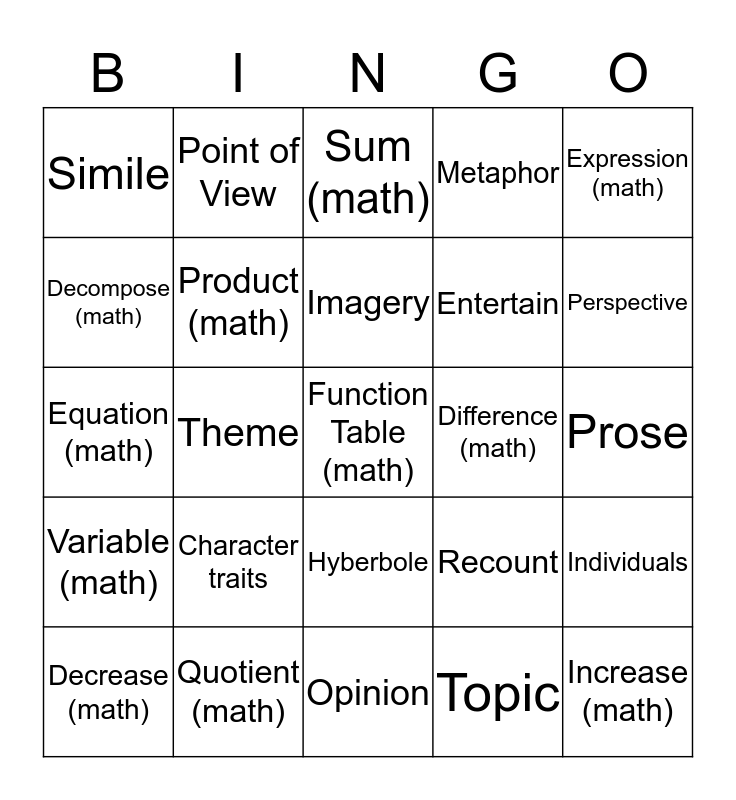# MCA BINGO Set TwoThis bingo card has a free space and 24 words: Character traits, Quotient (math), Imagery, Decrease (math), Expression (math), Simile, Decompose (math), Point of View, Difference (math), Hyberbole, Entertain, Equation (math), Recount, Individuals, Product (math), Topic, Prose, Sum (math), Perspective, Theme, Increase (math), Variable (math), Metaphor and Opinion.

## Play Online